# 6.4 Using the Modified Energy Transport Model

Fig. 6.10 shows the influence of the anisotropy parameter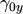on the output characteristics. By accounting for a reduced vertical electron temperature it is possible to reduce the spurious current decrease, but only to a certain degree and by assuming a fairly large anisotropy.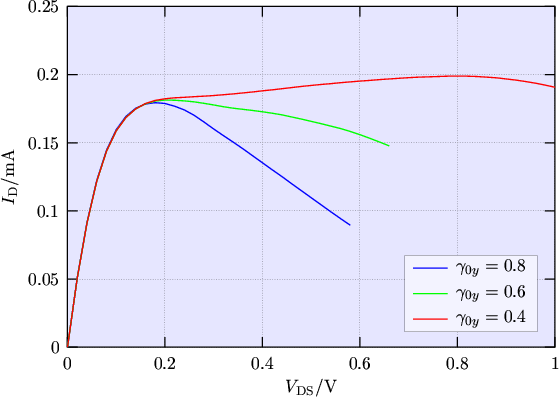By combining the modifications for an anisotropic temperature and a non-MAXWELLian closure relation the artificial current decrease is eliminated (Fig. 6.11). Parameter values roughly estimated from MC simulations can be used, for example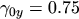and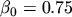. In the parameter range where the current drop is eliminated the output characteristics are found to be rather insensitive to the exact parameter values.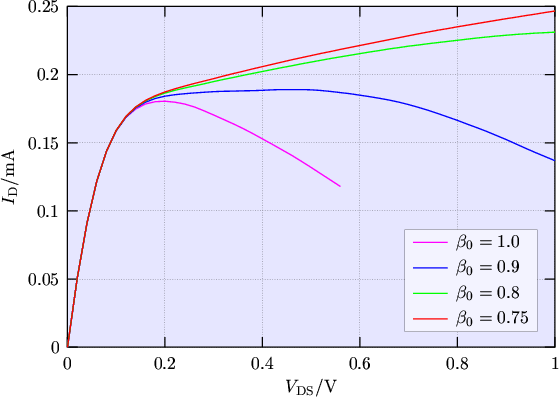When the modified model is applied to a body-contacted MOSFET, the difference in the output characteristic is only marginal compared to the standard energy transport model. For example using the values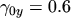andleads to a maximum deviation in the drain current of about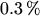compared to the standard energy transport model within the bias range.

Using the modified energy transport model good agreement of the electron concentration in vertical direction with Monte Carlo data is obtained (Fig. 6.12 and Fig. 6.13). This confirms that the correction of the SOI output characteristics obtained with the modified model is based on a corrected behavior of the electron distribution in the bulk.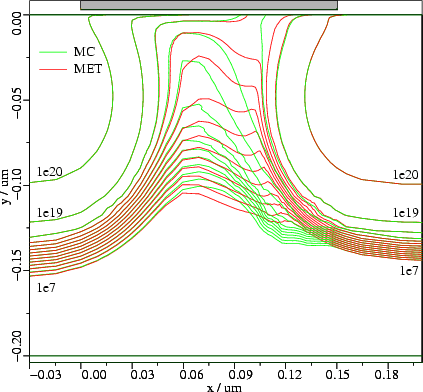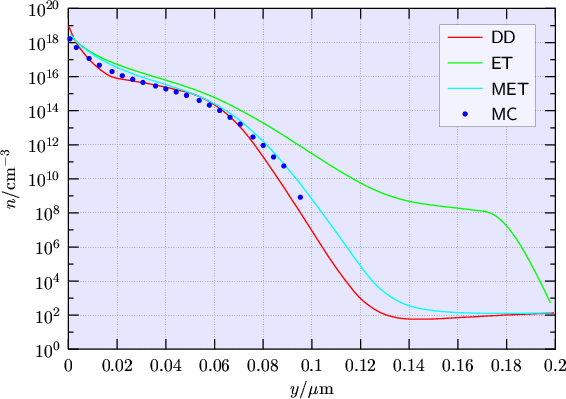M. Gritsch: Numerical Modeling of Silicon-on-Insulator MOSFETs PDF